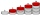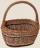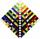# AVG of INT

What is the average of the integers from 9 throuht 52 inclusive?

Correct result:

x =  30.5

#### Solution:We would be pleased if you find an error in the word problem, spelling mistakes, or inaccuracies and send it to us. Thank you!Tips to related online calculators
Looking for help with calculating arithmetic mean?
Looking for a statistical calculator?

#### You need to know the following knowledge to solve this word math problem:

We encourage you to watch this tutorial video on this math problem:

## Next similar math problems:

• 75th percentile (quartille Q3)Find 75th percentile for 30,42,42,46,46,46,50,50,54
• SequenceBetween numbers 1 and 53 insert n members of the arithmetic sequence that its sum is 702.Combine like terms 4c+c-7c
• Median and modusRadka made 50 throws with a dice. The table saw fit individual dice's wall frequency: Wall Number: 1 2 3 4 5 6 frequency: 8 7 5 11 6 13 Calculate the modus and median of the wall numbers that Radka fell.
• SeatsSeats in the sport hall are organized so that each subsequent row has five more seats. First has 10 seats. How many seats are: a) in the eighth row b) in the eighteenth row
• Nineteenth memberFind the nineteenth member of the arithmetic sequence: a1=33 d=5 find a19
• AS sequenceIn an arithmetic sequence is given the difference d = -3 and a71 = 455. a) Determine the value of a62 b) Determine the sum of 71 members.
• AP - simpleDetermine the first nine elements of sequence if a10 = -1 and d = 4
• Insert 4Insert 3 arithmetic means between 3 and 63.Determine how many apples are in baskets when in the first basket are 4 apples, and in any other is 29 apples more than the previous, and we have eight baskets.
• What is 9What is the 15th term; x1=1.5, d=4.5?
• SequenceWrite the first 7 members of an arithmetic sequence: a1=-3, d=6.
• Sequence 2Write the first 5 members of an arithmetic sequence a11=-14, d=-1
• Sum of membersWhat is the sum of the first two members of the aritmetic progression if d = -4.3 and a3 = 7.5?
• TreesA certain species of tree grows an average of 0.5 cm per week. Write an equation for the sequence that represents the weekly height of this tree in centimeters if the measurements begin when the tree is 200 centimeters tall.
• SeriesYour task is express the sum of the following arithmetic series for n = 14: S(n) = 11 + 13 + 15 + 17 + ... + 2n+9 + 2n+11
• AverageIf the average(arithmetic mean) of three numbers x,y,z is 50. What is the average of there numbers (3x +10), (3y +10), (3z+10) ?